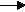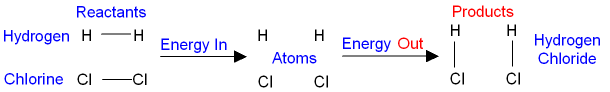gcsescience.com                                       25                                       gcsescience.com

Rates of Reaction

What are Bond Energy Calculations?

You can calculate the overall change in energy for a
reaction by using the values for the individual bond energies.

In the reaction between hydrogen and chlorine,

hydrogen + chlorinehydrogen chloride.
H2(g)  +     Cl2(g)2HCl(g)

The reactants are hydrogen and chlorine.
The product is hydrogen chloride.

You must put energy in to break the bonds in the reactants
before energy is given out by forming new bonds in the products.
The minimum amount of energy you need to put in
to make the reaction happen is called the activation energy.When the bonds between the hydrogen and the
chlorine molecules (the reactants) have been broken,
then both hydrogen and chlorine exist as atoms.

When an atom of hydrogen and chlorine react
with each other they make a new bond,
give energy out, and form the product hydrogen chloride.

What is the Bond Energy?

The bond energy is the amount of energy which
1) you must put in to break the bond,
or
2) is given out when the new bond is formed.

The table below shows some bond energies which are
given in kilojoules (thousand joules) per mole of bonds.

 Bond Bond Energy (in kJ per mole) H—H 436 Cl—Cl 242 H—Cl 431

In the reaction between hydrogen and chlorine, the big numbers
used to balance the equation (1H2   +   1Cl22HCl)
tell you how many moles there are of reactant and product.

You must break
1 mole of HH bonds and 1 mole of ClCl bonds.
The amount of energy needed (from the above table) is
436 + 242 kJ
= 678 kJ.

When 2 moles of HCl bonds are formed,
the amount of energy given out is
2 x 431 kJ
= 862 kJ.

The difference between the two amounts of energy
(reactants minus products) is the overall
amount of energy given out per mole of reactants.
678 - 862 kJ = -184 kJ.

The change in energy during a reaction is given
the symbol ΔH.
In the reaction between hydrogen and chlorine,

ΔH = -184 kJ per mole.

For an exothermic reaction, ΔH is negative.

gcsescience.com        The Periodic Table        Index        Energy Quiz        gcsescience.com